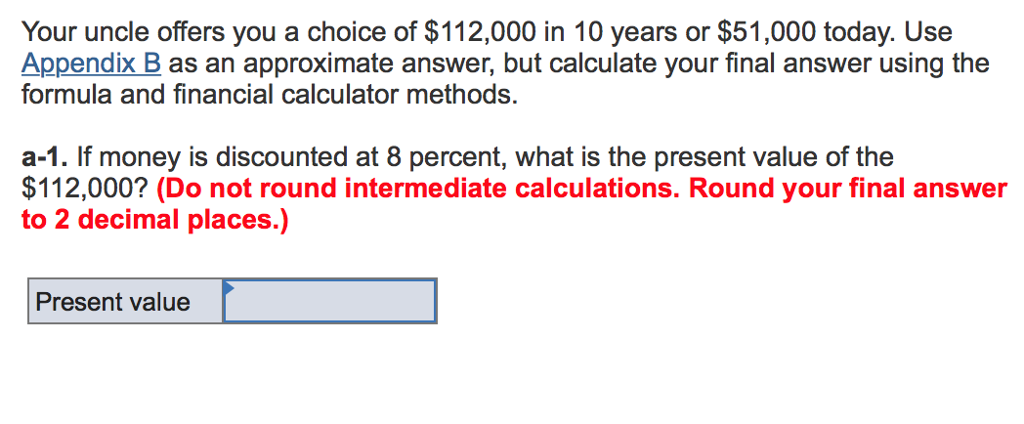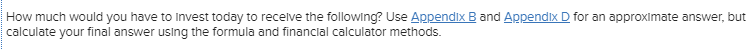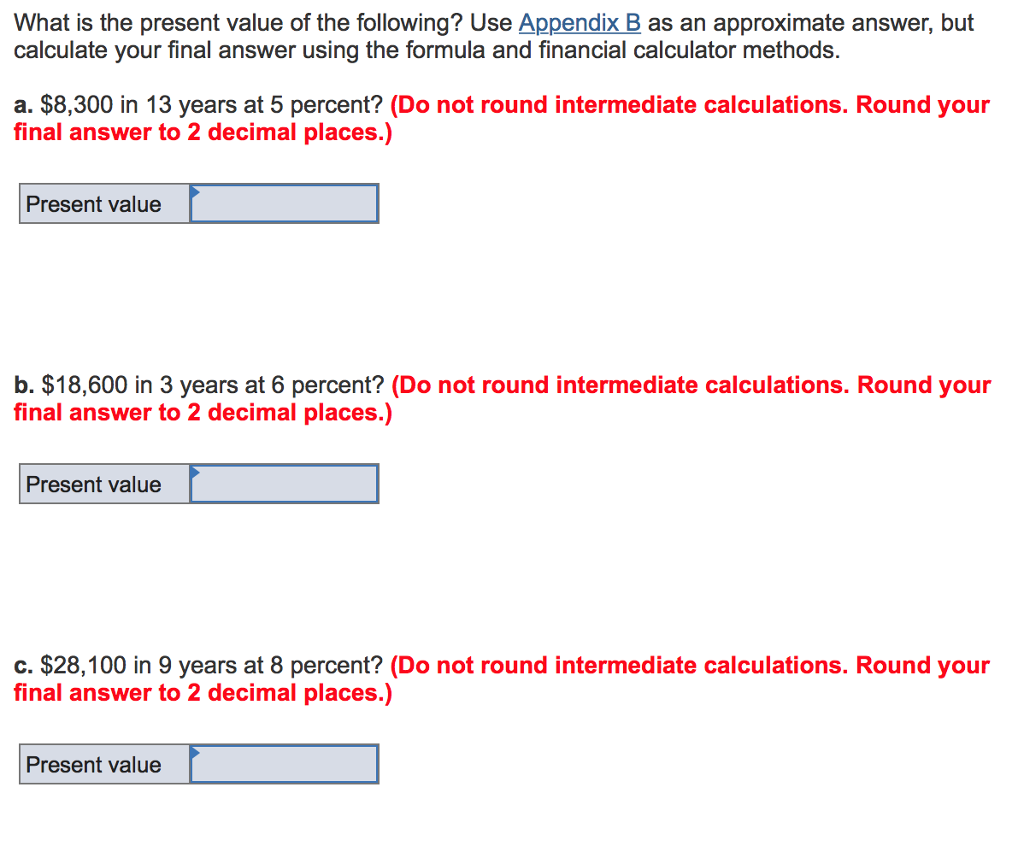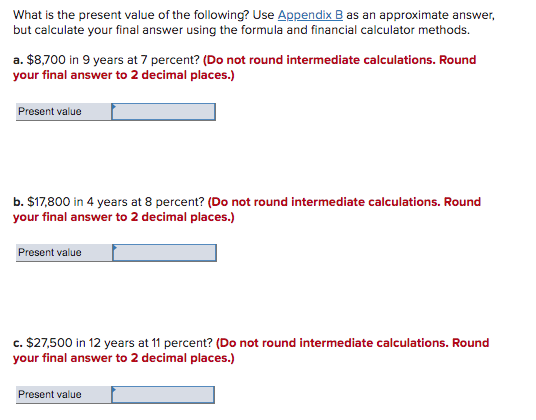# Your uncle offers you a choice of \$112,000 in 10 years or \$51,000 today. Use Appendix...Your uncle offers you a choice of \$112,000 in 10 years or \$51,000 today. Use Appendix B as an approximate answer, but calculate your final answer using the formula and financial calculator methods. a-1. If money is discounted at 8 percent, what is the present value of the \$112,000? (Do not round intermediate calculations. Round your final answer to 2 decimal places.) Present value

Amount today will be compared with the present value of amount to be received later

Present Value = Amount*PVF(8%, 10 years)

= 112,000*0.463

= \$51,856

Hence, it is better to receive \$112,000 10 years later.

#### Earn Coin

Coins can be redeemed for fabulous gifts.

Similar Homework Help Questions
• ### Your father offers you a choice of \$125,000 in 11 years or \$45,500 today. Use Appendix B as an ap...

Your father offers you a choice of \$125,000 in 11 years or \$45,500 today. Use Appendix B as an approximate answer, but calculate your final answer using the formula and financial calculator methods. a-1. If money is discounted at 8 percent, what is the present value of the \$125,000? (Do not round intermediate calculations. Round your final answer to 2 decimal places.)    a-2. Which offer should you choose?    \$45,500 today \$125,000 in 11 years b-1. Now assume the offer is...

• ### How much would you have to invest today to receive the following? Use Appendix B or...

How much would you have to invest today to receive the following? Use Appendix B or Appendix D for an approximate answer, but calculate your final answer using the formula and financial calculator methods.    a. \$15,250 in 11 years at 7 percent. (Do not round intermediate calculations. Round your final answer to 2 decimal places.)    b. \$19,600 in 18 years at 11 percent. (Do not round intermediate calculations. Round your final answer to 2 decimal places.)    c....

• ### How much would you have to Invest today to receive the following? Use Appendix B and Appendix D for an approximate ans...How much would you have to Invest today to receive the following? Use Appendix B and Appendix D for an approximate answer, but calculate your final answer using the formula and financial calculator methods. \$7,800 each year for 20 years at 6 percent. (Do not round Intermediate calculations. Round your final answer to 2 decimal places.) Present value 553,000 each year for 25 years at 12 percent. (Do not round Intermediate calculations. Round your final answer to 2 decimal places.)...

• ### Your grandfather has offered you a choice of one of the three following alternatives: \$5,500 now; \$1,250 a year for five years; or \$17,000 at the end of five years. Use Appendix B and Appendix D for an approximate answer, but calculate your final answer u

Your grandfather has offered you a choice of one of the three following alternatives: \$5,500 now; \$1,250 a year for five years; or \$17,000 at the end of five years. Use Appendix B and Appendix D for an approximate answer, but calculate your final answer using the formula and financial calculator methods.a-1. Assuming you could earn 6 percent annually, compute the present value of each alternative: (Do not round intermediate calculations. Round your final answers to 2 decimal places.)1250 ->    Present value = _________  a-2. If you...

• ### What is the present value of the following? Use Appendix B as an approximate answer, but...What is the present value of the following? Use Appendix B as an approximate answer, but calculate your final answer using the formula and financial calculator methods. a. \$8,300 in 13 years at 5 percent? (Do not round intermediate calculations. Round your final answer to 2 decimal places.) Present value b. \$18,600 in 3 years at 6 percent? (Do not round intermediate calculations. Round your final answer to 2 decimal places.) Present value c. \$28,100 in 9 years at 8...

• ### What is the present value of the following? Use Appendix B as an approximate answer, but...What is the present value of the following? Use Appendix B as an approximate answer, but calculate your final answer using the formula and financial calculator methods. a. \$8,700 in 9 years at 7 percent? (Do not round intermediate calculations. Round your final answer to 2 decimal places.) Present value b. \$17,800 in 4 years at 8 percent? (Do not round intermediate calculations. Round your final answer to 2 decimal places.) Present value C. \$27,500 in 12 years at 11...

• ### Your grandfather has offered you a choice of one of the three following alternatives:

Your grandfather has offered you a choice of one of the three following alternatives: \$5,500 now; \$1,250 a year for five years; or \$17,000 at the end of five years. Use Appendix B and Appendix D for an approximate answer, but calculate your final answer uYour grandfather has offered you a choice of one of the three following alternatives: \$5,500 now; \$1,250 a year for five years; or \$17,000 at the end of five years. Use Appendix B and Appendix D for an approximate...

• ### Your uncle offers you a choice of \$30,000 in 50 years or \$95 today

Your uncle offers you a choice of \$30,000 in 50 years or \$95 today. If money is discounted at 12 percent, which should you choose? Can anyone tell me if this is right and if not what am I doing wrong. 30,000 x 0.003= \$90Option 2:PV = \$95

• ### What is the present value of the following? Use Appendix B as an approximate answer, but...

What is the present value of the following? Use Appendix B as an approximate answer, but calculate your final answer using the formula and financial calculator methods. a. \$7,800 in 6 years at 10 percent? (Do not round intermediate calculations. Round your final answer to 2 decimal places.)    b. \$16,500 in 3 years at 7 percent? (Do not round intermediate calculations. Round your final answer to 2 decimal places.)    c. \$25,700 in 9 years at 8 percent? (Do...

• ### What is the present value of the following? Use Appendix B as an approximate answer, but...

What is the present value of the following? Use Appendix B as an approximate answer, but calculate your final answer using the formula and financial calculator methods. a. \$8,600 in 5 years at 10 percent? (Do not round intermediate calculations. Round your final answer to 2 decimal places.)    b. \$17,600 in 3 years at 6 percent? (Do not round intermediate calculations. Round your final answer to 2 decimal places.) c. \$27,100 in 9 years at 7 percent? (Do not...